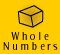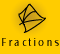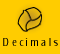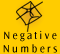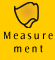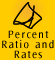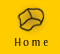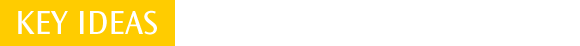| Benchmark percents | Percent increases and decreases |
Equivalent ratios | Quick quiz |

Benchmark percents

Some equivalent percents, common fractions and decimal fractions we should easily be able to recall are: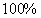= 1.0 = 1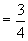= 0.75= 0.5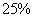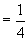= 0.25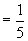= 0.2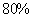= 0.8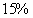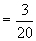= 0.15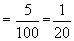= 0.05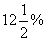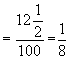= 0.125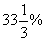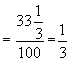= 0.33 (rounded to 2 decimal places)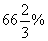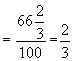= 0.67 (rounded to 2 decimal places)

Knowing these values which are very commonly used in our daily lives means that we can quickly and easily find the answer to many percent problems with a minimum of extended working out.

For example, if I want to find out what percent of the pizza has been eaten and I know that there is 1/4 of the pizza left, then I know 75% has been eaten.

For example, if I know that there is a 33 1/3% increase in wheat production I also know that wheat production has increased by one third.

Estimation

We can apply our knowledge of benchmarks to making estimations. When using percents in every day situations an estimation will usually suit our needs. Estimations are also useful when we want to have a fair idea of the answer expected to a problem before working it out accurately.

For example,

 47% is just under half 70% is just over two thirds 19% is about one fifth 69% of 311 is about two thirds of 311 and is a bit over 200 48% of 820 is just under 410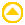Percent increases and decreases

This subtopic has been included prior to Percent Examples because it introduces key ideas about percent in situations with which most of us are familiar. Please note, many more percent problems and explanations are included in the Percent Examples section.

Example 1:

If the price of a jacket was \$200 and has now been reduced to \$100, what is the percent decrease?

What did you answer to this question? Did you answer 100% or 50%?

 Thinking If the jacket was originally \$200 and it has been reduced to \$100 then it has been marked down by half of its original price (\$100 out of \$200), it has been reduced by 50%. (If you thought that the price was reduced by 100% then this would mean that the jacket was actually marked down to nothing. To check your answer, ask '100% of what?' 100% of the original price of \$200 is \$200, so \$200 reduced by \$200 leaves nothing! The answer cannot be 100%)

Percent increases and decreases are always taken as a fraction of the original amount, in this case, price.

The dual-scale number line model, discussed earlier in Meaning and Models: Percent Models, can be a clear way of illustrating the problem.

 We have labelled the percent scale on the left hand side of the number line. We have labelled the right hand side with the corresponding dollar amounts in the problem.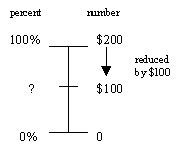We know that \$200 is the original price, ie. the 100% price. We know that \$100 is the reduced price. We need to find out what percent of the original price is the reduced price. We have put a '?' next to the information we need to find out. Now using this model we can see by equivalent fractions that, ?/100 =\$100/\$200 and that the discount is 50%. This model also clearly shows that the answer could not be 100%, since 100% is \$200.

Example 2:

If the price of a TV went up from \$200 to \$300, by what percent did it increase?

 Thinking The TV was originally priced at \$200. It was increased to \$300, which means it was increased by half of the original price. \$200 increased to \$300 is an increase of \$100 out of \$200 which is 50%. The new price is 150% of the old price.

People commonly make errors when working with percent increases and decreases. The important question to ask is 'the percent of what?'.

Everyday usage of percent
In order to use percent in our everyday lives we need to understand the common expressions we use to talk about them.

'Gone up by 20%' means an increase of 20% of the original quantity.

The movie below shows two different ways to calculate an increased quantity.

Example 3: Movie - calculating an increased quantity.

Since the recent rains the production of cotton has gone up by 20%. If a farmer produced 300 tonnes prior to the rain, how much will he produce now?

We have used both the methods shown below to find the increased quantity of 20%.

 new amount = old amount + (20% of old amount), OR new amount = old amount x (1.20) NOTE: We have expressed 1.20 as 1.2 because it is easier to read on the screen. '1.20' is the same as '1.2'.

'Gone up to 110%' means an increase of 10%.

(NOTE: This expression can easily be confused with 'gone up by 110%' which means an increase of 110%!)

Example 4: Movie - calculating an increased quantity of 10%.The opposition was quoted as saying 'since the introduction of the GST books have gone up to 110% of their previous price....'. If the total cost of 250 new books for the library was \$3500 pre-GST, how much would the cost be now?

As we are still talking about an increase we have used the same methods to find the new amount.

 new amount = old amount + (10% x old amount), OR new amount = old amount x (1.10)

'Went down by 20%' or 'discounted by 20%' or 'marked down by 20%' are common terms used when we are talking about money. All of these terms mean a decrease of 20%.

Example 5: Movie - calculating a decreased quantity of 20%

A shoe store is having a sale where everything has been discounted by 20%. At the sale, Tan bought a pair of shoes for \$40. What was the original price of the shoes?

We also could have used the following methods, but as we knew the 'new amount' and were looking for the 'old amount' we would have had to transpose the equations to find the 'old amount'.

 New amount = old amount - (20% x old amount), OR new amount = old amount x 0.80, OR by transposing this equation, old amount = new amount / 0.80

'Went down to 75%' or 'marked down to 75%' means a decrease of 25%.

(NOTE: It is easy to confuse 'went down to 75%' with 'went down by 75%' - they do not mean the same thing!)

Example 6: Movie - calculating a decreased quantity of 75%

Since the increase in competition amongst telecommunications companies, the price of international phone calls has decreased by 25%.
If the average cost per month of international calls in our household was \$400, what would it be now with the reduced call costs?

 new amount = old amount - 25% of old amount, OR new amount = old amount x (1 - 0.25) new amount = old amount x 0.75

Less common percent increases and decreases

Although we have covered percent increases and decreases we most commonly come across, it is important to still be able to work with more complicated numbers.

Example 7:

Council rates are set to increase by 7.5% this year. If my rates were \$815 last year, what will they be this year?

Setting Out
 new amount = old amount x 1.075 = 815 x 1.075 = 876.125 Answer is \$876.13 (rounded to the nearest cent)
Thinking

I need to calculate the increased amount of 7.5% on \$815. To do this I multiply the old amount by 1 plus the percent increase. The percent increase expressed as a decimal is 0.075. Therefore I will multiply the old amount by 1.075.

NOTE: A VERY common error is to express 7.5% as 0.75. To avoid this error, remember that 7.5% is 7.5 divided by 100 (7.5/100). which is 0.075 (A bit more than 7 hundredths 0.07).

More examples are shown in the table below.

 % change Expressed as a fraction and as a decimal Calculation an increase of 8.3% 8.3/100, 0.083 new amount = old amount x 1.083 a decrease of 0.5% 0.5/100, 0.005 new amount = old amount x (1 - 0.005) new amount = old amount x 0.995 an increase of 27.5% 27.5/100, 0.275 new amount = old amount x 1.275

Example 8:

It has been forecasted that property values are set to rise 3% per year over the next 3 years. If Lionel's property is worth \$250 000 now, what will it be worth at the end of the next 3 years?

Setting Out
Thinking
 Year 1 new amount = \$250 000 x 1.03 = \$257 500 Year 2 new amount = \$257 500 x 1.03 = \$265 225 Year 3 new amount = \$265 225 x 1.03 = \$273 181.75

We know that to calculate an increase of 3% we use the following formula,

new amount = old amount x 1.03

(we multiply by 1.03 because we have an increase of 3/100 or 0.03)

This will calculate our increase for the first year.

As there is the same percent increase in the second year we can also multiply the amount at the end of the first year by 1.03

As there is also the same percent increase in the third year we can multiply the amount at the end of the second year by 1.03.

The property has increased in value to \$273 181.75 by the end of the third year.

Alternative method Thinking
 new amount = \$250 000 x 1.03 x 1.03 x 1.03 = \$273 181.75

We can see from above that for each year of increase we multiplied the new amount by 1.03. We could have worked out this problem in one step by multiplying the original amount by 1.03, 3 times. Try it on your calculator!

Value for Money

A common application of percent is comparing value for money.

Example 9:

Which video recorder is the best buy? Find the percent discount of each video and determine which has the biggest percent discount?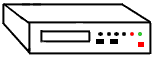\$190, save \$15\$320 save \$40\$225, save \$30\$375, save \$40\$495, save \$60\$550, save \$75

The percent discount for each item is,

 15/205x100 = 7.32% 40/360x100=11.11% 30/255x100=11.76% 40/415x100=9.64% 60/555x100=10.81% 75/625x100=12%

The video recorder with a discounted price of \$550 has the largest percent discount (although not by much) of 12%.

Equivalent ratios

Example 10:

Jay loves white chocolate mud cake. He is eating a piece of chocolate mud cake which contains 1 part white chocolate to 2 parts milk chocolate when he sees another chocolate mud cake in a shop window which is advertised as containing 6 parts white chocolate to 12 parts milk chocolate. Does the cake in the window contain a higher ratio of white to milk chocolate?

 Thinking Jay's piece of cake has a ratio of white to milk chocolate of 1 : 2. The cake in the window has a ratio of white to milk chocolate of 6 : 12. We can see that 6 : 12 is the same ratio as 1 : 2 because if there are 6 parts of white to 12 parts of milk, then there must be 1 (smaller) part of white to 2 parts of milk chocolate. 6:12 can be simplified to 1 : 2 (by dividing both terms by 6). Therefore the cakes contain the same ratio of white to milk chocolate.

In example 4 in Meaning and Models, Ratio we saw that the ratio of 1 : 4 is equivalent to 2 : 8. If we mixed blue to white paint in the ratio of 1 : 4 and then mixed some more paint later in the ratio 2 : 8 we would get exactly the same colour.

Realising that ratios have many equivalent ratios is crucial to using ratios efficiently.

Simplifying Ratios

Example 11: Simplify the following:

 Example Thinking boys : girls = 6 : 12 Both numbers can be divided by 6, so 6 : 12 = 1 : 2 hot chocolate : coffee : tea = 3 : 27 : 18 All numbers can be divided by 3, so 3 : 27 : 18 = 1 : 9 : 6 blue : red : yellow = 16 : 12 : 20 All numbers can be divided by 4, so 16 : 12 : 20 = 4 : 3 : 5 students : computers = 28 : 9 There is no whole number which will divide both numbers exactly, however we can divide both numbers by 2 to give, 14 : 4.5

The total number of parts

Another important aspect of ratios is understanding how many parts there are in total. We often want to compare the ratio of parts but we may also want to know the ratio of parts compared to all the parts in total.

Example 12:

If red to yellow paint has been mixed in the ratio of 1 : 3, this means there is 1 part red to 3 parts yellow and that there are 4 parts in total.

One ratio can give us a lot of information. If I know that the ratio of red to yellow paint is 1 : 3 I also know:

 the ratio of yellow to red is 3 : 1 the ratio of red to the total number of parts is 1 : 4 the ratio of yellow to the total number of parts is 3 : 4 We could also say we have 1/4 red and 3/4 yellow. We could also say that we have 25% red and 75% yellow.

We can see that when we are comparing parts to the whole we can do so using ratios, fractions or percents.

 Example 13: Equivalent ratios.. Concrete is mixed in the ratio of 3 : 2 : 1 = screenings : sand : cement. Work out the quantity of each amount required to make a concrete mix. (a) If I add 6 shovels of sand, how much screenings and cement will I need? (b) If I add 12 shovels of screenings,how much sand and cement will I need to add? (c) If I add 4 bags of cement, how much sand and screenings will I need to add? (d) If I add 3 wheelbarrows of sand, how many barrows of screenings and cement will I need?

 Thinking (a) If I need to mix screenings : sand : cement in the ratio of 3 : 2 : 1 and I have 6 shovels of sand then this represents 2 parts. So 1 part would be 3 shovels. So the amount of screenings would be 3 x 1 part = 3 x 3 shovels = 9 shovels. The amount of cement would be 1 x 1 part = 1 x 3 shovels = 3 shovels. So the amounts are 9, 6 and 3 respectively. (b) Now I have 12 shovels of screenings. This represents 3 parts in the ratio of screenings 3 : sand 2 : concrete 1. Therefore 1 part is four shovels. So the amount of sand is 2 x 4 shovels = 8 shovels. The amount of concrete required is only 1 part so this is 4 shovels. 3 : 2 : 1 = 12 : 8 : 4 (c) I now have 4 bags of cement. There is only 1 part cement to 3 parts screenings and 2 parts sand required, so 4 bags of cement is equivalent to 1 part. To make the concrete mix in this case, I need 12 bags of screenings and 8 bags of sand. All the bags must be the same size. 3 : 2 : 1 = 12 : 8 : 4 (d) Now I have 3 wheelbarrows of sand. There are 2 parts sand in the concrete mix ratio, so 3 wheelbarrows represents 2 parts, and 1.5 wheelbarrows represents 1 part. Therefore 3 x 1.5 wheelbarrows of screenings are required and 1 x 1.5 wheelbarrows of cement is required. 3 : 2 : 1 = 4.5 : 3 : 1.5

 NOTE: This ratio of 3 : 2 : 1 = screenings : sand : cement will apply for any measure of volume, such as a bucket, bag, shovel, spade etc. It is not a ratio of a measure of weight. We can see that this makes sense since the weights and volumes of sand, screenings and cement would vary considerably. Volume is an easier measure to use when concreting.Quick quiz

 1. Express the following percents as fractions and decimals: a) 33 1/3% b) 231% c) 0.03% d) 75% e) 12.5% 2. Calculate the new price if the following items were marked down by 20%: a) a bottle of juice at \$2.30 b) a computer at \$1 900 c) a litre of oil at \$6.50 d) a movie ticket at \$11 e) a new car at \$40 000 f) a CD at \$30 3. Calculate the new price if the following items went up by 15%: a) a bottle of juice at \$2.30 b) a computer at \$1 900 c) a litre of oil at \$6.50 d) a movie ticket at \$11 e) a new car at \$40 000 f) a CD at \$30 4. Estimate the following percentages (DO NOT WORK THEM OUT ON YOUR CALCULATOR): a) Estimate 9% of 70 b) Estimate 48.5% of 120 c) Estimate 24% of 200 d) Estimate 0.5% of 100 e) Estimate 98% of 163 f) Estimate 19% of 5000 g) Estimate 47% of 820 h) Estimate 69% of 8960 5. Find at least one equivalent ratio for the following: a) pens : pencils = 6 : 12 b) blue eyes: brown eyes = 1 : 3 c) teachers : students = 2 : 7 d) water : orange juice : softdrink = 7 : 22 : 35 e) A : B : C : D = 3 : 9 : 24 : 36 f) width : length : height = 5 : 10 : 26 6. Simplify the following ratios into lowest terms: a) X : Y : Z = 14 : 6 : 28 b) cm on map: cm on ground = 2 : 50 000 c) L : W : H = 12 : 4 : 48 d) inches on ground : inches on map = 30 000 : 3